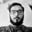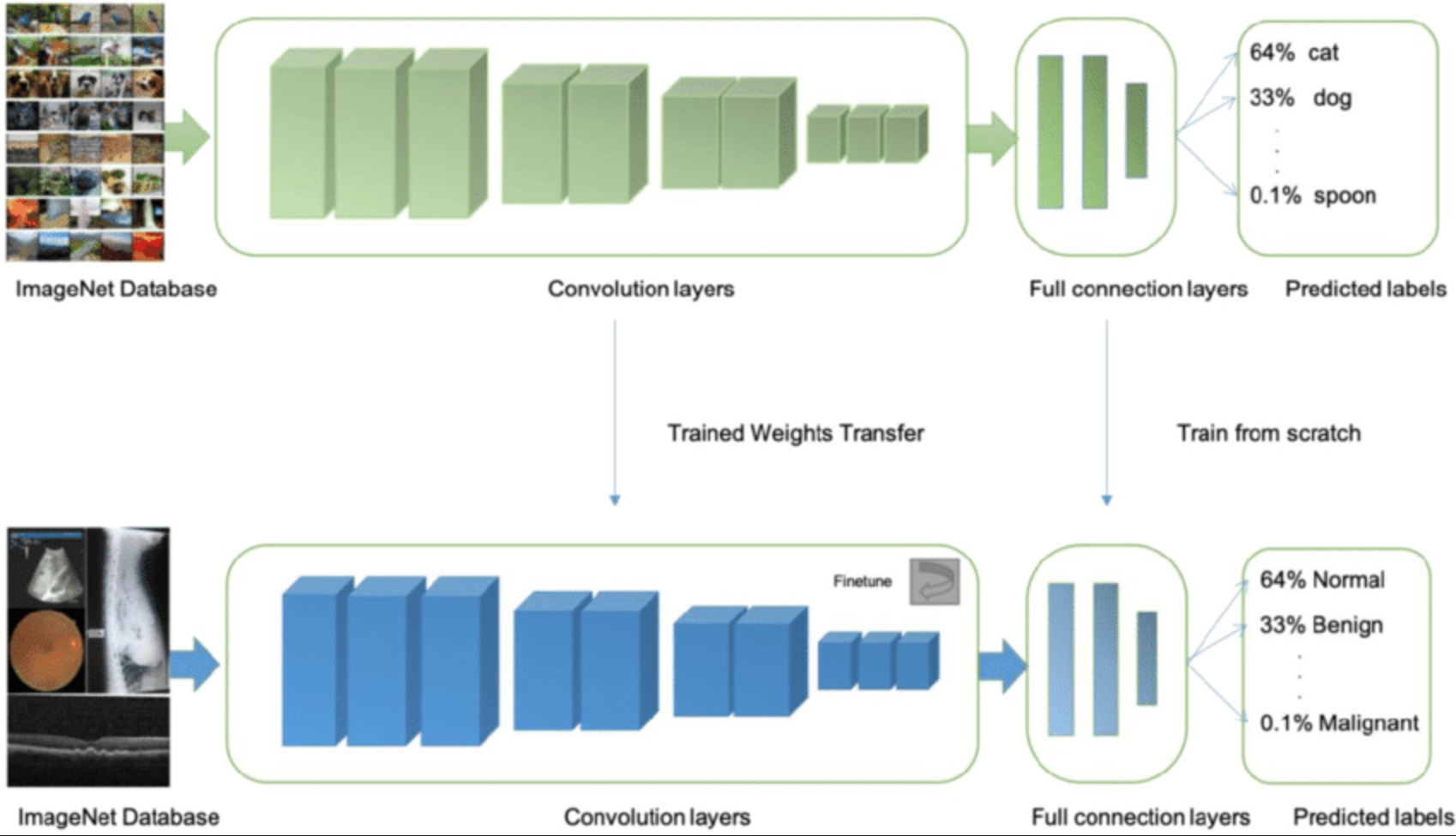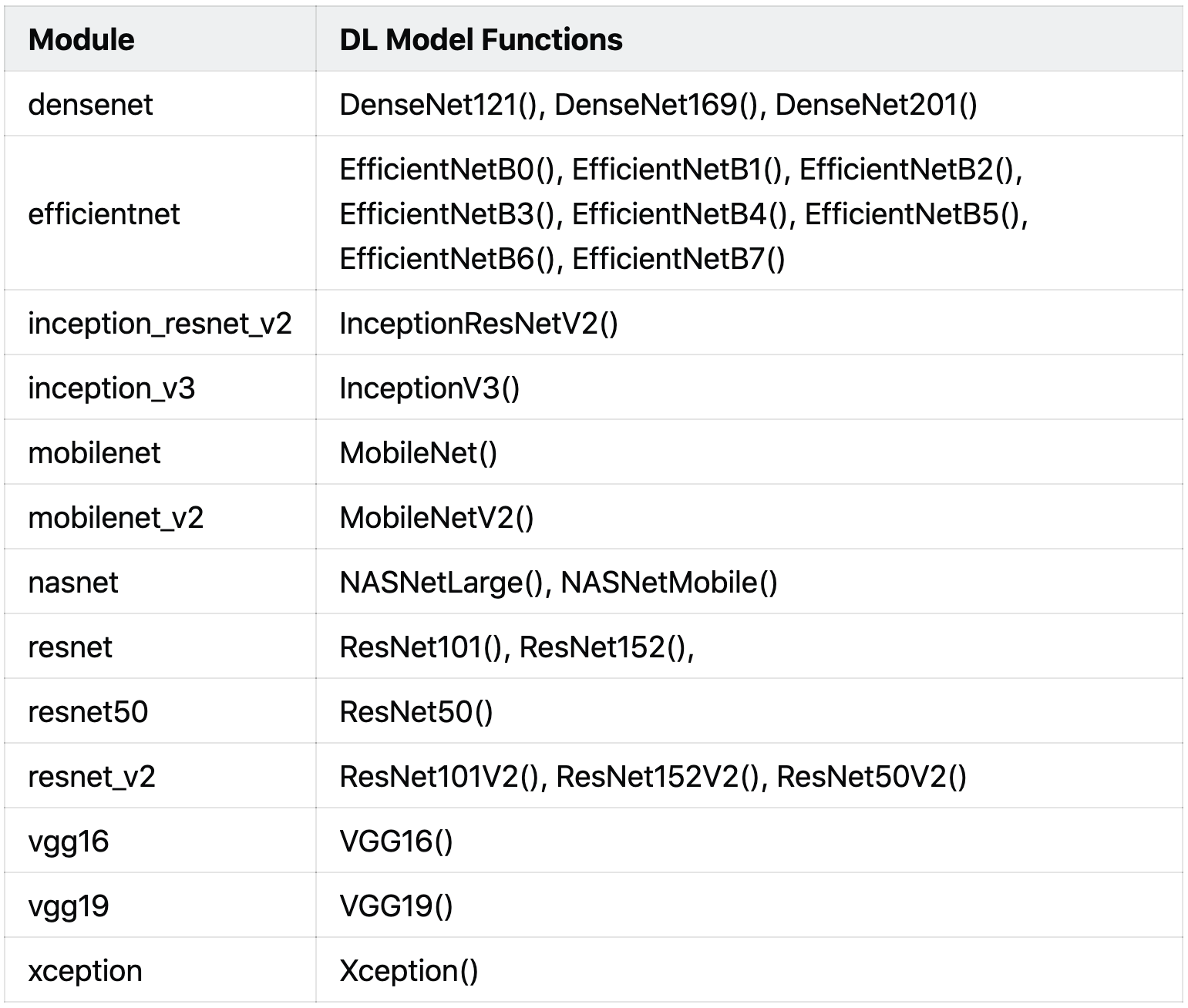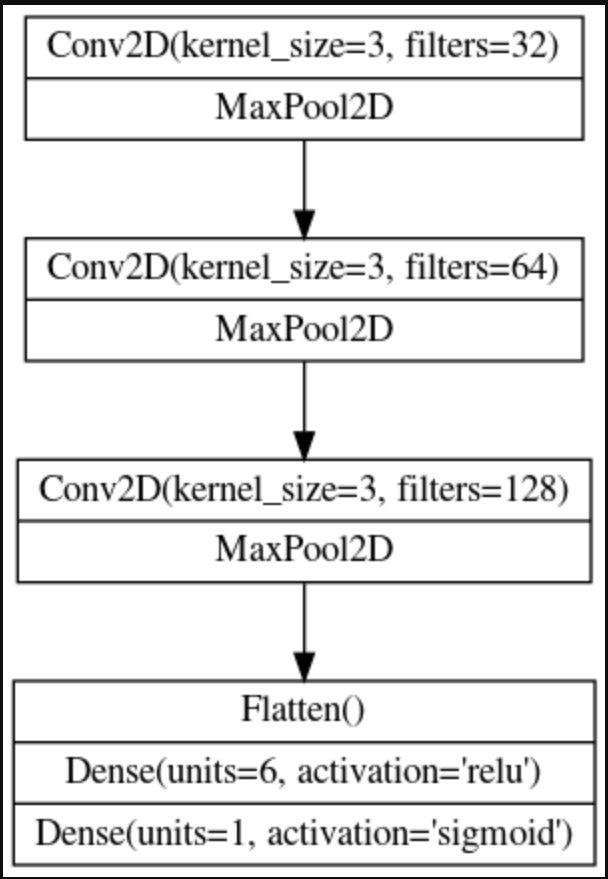Related Tags

machine learning
beginners
python
community creator

# Pre-trained models for Transfer Learning in KerasAman Anand

TensorFlow is one of the most highly used libraries for Machine Learning. It also has built-in support for Keras. We can easily call functions related to Keras using the tf.keras module.

Computer Vision is one of the most interesting branches of Machine Learning. The ImageNet dataset was the turning point for Computer Vision researchers as it provided a large set of images for Object detection. Now, ImageNet is a benchmark for testing the accuracy of Image Classification and Object Detection deep learning models.

Transfer Learning is also one of the major developments in the case of Deep Learning for Object Detection. In Transfer Learning, we take a pre-trained model performing classification on a dataset and apply the same model to another set of classification tasks by optimizing the hyper-parameters a little bit.

Transfer Learning has two benefits:

• It requires less time to train a model as it has already been trained on a different task
• It can be used for tasks with have smaller datasets as the model is already trained on a larger dataset, and so the weights are transferred to the new taskThe above illustration of Transfer Learning shows a model trained for object detection (like Cat,Dog,etc.) being used again for Cancer Detection by transferring weights.

The Tensorflow Keras module has a lot of pre-trained models that can be used for transfer learning. Details about this can be found here. The tf.keras.applications module contains these models.

A list of modules and functions for calling Deep learning model architectures present in the tf.keras.applications module is given below:We write models in TensorFlow as per the example below:

import tensorflow.keras as keras

model = keras.Sequential([

# First Convolutional Block
layers.Conv2D(filters=32, kernel_size=5, activation="relu", padding='same',input_shape=[128, 128, 3]),
layers.MaxPool2D(),

# Second Convolutional Block
layers.MaxPool2D(),

# Third Convolutional Block
layers.MaxPool2D(),

layers.Flatten(),
layers.Dense(units=6, activation="relu"),
layers.Dense(units=1, activation="sigmoid"),
])


The structure of this Deep Learning model is as follows:In the same way, we can call the Xception() function from the tf.keras.applications module to add the pre-trained model to our architecture. This model is pre-trained, so we are taking the weights from the previous dataset or task (‘imagenet’) into our model and not training it again. Hence, the parameter trainable is set to False, a globalaveragepooling layer is used, and softmax is used for multiclass classification.

In the case of binary classification, the activation function must be sigmoid.

pretrained_model = tf.keras.applications.Xception(
weights='imagenet',
include_top=False ,
input_shape=[*IMAGE_SIZE, 3]
)
pretrained_model.trainable = False

model = tf.keras.Sequential([
pretrained_model, tf.keras.layers.GlobalAveragePooling2D(), tf.keras.layers.Dense(len(CLASSES), activation='softmax')
])


We can use all the different models in the same way by just changing the functions.

RELATED TAGS

machine learning
beginners
python
community creator

CONTRIBUTORAman Anand